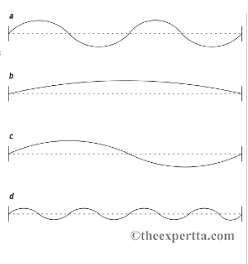# Problem:  Using special techniques called string harmonics (or "flageolet tones"), stringed instruments can produce the first few overtones of the harmonic series. While a violinist is playing some of these harmonics for us, we take a picture of the vibrating string (see figures). Using an oscilliscope, we find the violinist plays a note with frequency f = 830 Hz in figure (a).A) The string length of a violin is about L = 33 cm. What is the wavelength of the standing wave in figure (a) in meters?B) the fundamental frequency is the lowest frequency that a string can vibrate at (see figure (b). What is the fundamental frequency for our violin in Hz?C) In terms of the fundamental frequency f1, what is the frequency of the note that the violinist is playing in figure (c)?D) Write a general expression for the frequency for any note that the violinist can play in this manner, in terms of fundamental frequency f1, and the number of antinodes on the standing wave A.E) What is the frequency, in hertz, of the note the violinist is playing in figure (d)?

###### FREE Expert Solution

(a)

The string has two wavelengths.

2λ = L

λ = L/2 = 33/2 = 16.5 cm(1m/100cm) = 0.165m

81% (426 ratings)###### Problem Details

Using special techniques called string harmonics (or "flageolet tones"), stringed instruments can produce the first few overtones of the harmonic series. While a violinist is playing some of these harmonics for us, we take a picture of the vibrating string (see figures). Using an oscilliscope, we find the violinist plays a note with frequency f = 830 Hz in figure (a).A) The string length of a violin is about L = 33 cm. What is the wavelength of the standing wave in figure (a) in meters?

B) the fundamental frequency is the lowest frequency that a string can vibrate at (see figure (b). What is the fundamental frequency for our violin in Hz?

C) In terms of the fundamental frequency f1, what is the frequency of the note that the violinist is playing in figure (c)?

D) Write a general expression for the frequency for any note that the violinist can play in this manner, in terms of fundamental frequency f1, and the number of antinodes on the standing wave A.

E) What is the frequency, in hertz, of the note the violinist is playing in figure (d)?